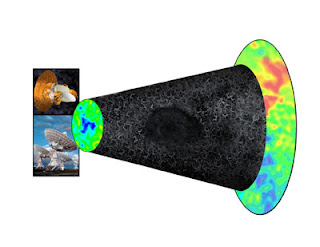## Tuesday, March 24, 2009

### Voiding the issueThere's a nice article by Timothy Clifton and Pablo Ferreira in the April issue of Scientific American, on the possibility that we inhabit a comparative void in the universe.

A decade ago, observations of distant supernovae found that for a given redshift, the supernovae were dimmer than expected. This suggested that the supernovae must be further away than expected, which, in turn, entailed that their light must have taken longer to reach us than expected for their redshift. Assuming a spatially homogeneous distribution of matter, this implied that the universe must have taken longer to reach its present size than it would have if the expansion rate had never been slower in the past. Hence, it was concluded that the expansion rate must be getting faster.

This apparent acceleration in the expansion of the universe has typically been explained by postulating the presence of gravitationally repulsive 'dark energy'. However, it could also potentially be explained if we inhabit a region of lower-than-average matter density in the universe. Regions of lower-than-average density expand faster than denser regions, hence the light entering an under-dense region will experience an increasing redshift which mimics the effect of a universe whose expansion is accelerating. As Clifton and Ferreira put it:

Light traveling a given distance is redshifted by less than it would be if the whole universe expanded at our local rate. Conversely, to achieve a certain redshift in such a universe, the light has to travel a greater distance than it would in a uniformly expanding universe, in which case the supernova has to be farther away and therefore appear dimmer.

Whilst the supernovae observations which support the notion of accelerative expansion extend out to billions of light years, observations of the distribution of galaxies only extend out to hundreds of millions of light years. Hence, although the distribution of galaxies might look homogeneous on the length-scales hitherto observed, it may be that the universe is inhomogeneous on longer length-scales. This potentially challenges the Copernican Principle, which assumes that our perspective upon the universe is typical.

Clifton and Ferreira also provide an accurate, concise explanation of an intriguing foundational problem in general relativistic cosmology, which might explain the apparent observation of accelerative expansion:

Another possibility is that dark energy is an artifact of the mathematical approximations that cosmologists routinely use. To calculate the cosmic expansion rate, we typically count up how much matter a region of space contains, divide by the volume of the region and arrive at the average energy density. We then insert this average density into Einstein's equations for gravity and determine the averaged expansion rate of the universe. Although the density varies from place to place, we treat this scatter as small fluctuations about the overall average.

The problem is that solving Einstein's equations for an averaged matter distribution is not the same as solving for the real matter distribution and then averaging the resulting geometry. In other words, we average and then solve, when really we should solve and then average.